Queries for Integer partitions: search statistic / browse statistics / browse maps from / browse maps to

# Definition & Example

• An integer partition $\lambda$ of $n \in \mathbb{N}_+$ is a sequence $\lambda = (\lambda_1,\ldots,\lambda_k)$ such that $\lambda_i \in \mathbb{N}_{+}$, $\lambda_1 \geq \lambda_2 \geq \dots \geq \lambda_k$ and $\sum_{1 \leq i \leq k} \lambda_i = n$.

• We write $\lambda \vdash n$ if $\lambda$ is a partition of $n$, and denote the set of integer partitions of $n$ by $\mathbf{P}_n$.

 the 7 Integer partitions of size 5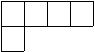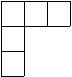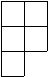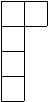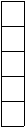[4,1] [3,2] [3,1,1] [2,2,1] [2,1,1,1] [1,1,1,1,1]

• Integer partitions are graphically represented by their Ferrers diagram (or Young diagram) as a collection of boxes.

• The number of integer partitions $p(n) = |\mathbf{P}_n|$ is A000041, and the number of integer partitions into distinct parts is A000009. Its generating function is

$$\displaystyle \sum_{n=0}^\infty p(n) x^n = \prod_{k=1}^\infty \frac{1}{1-x^k}.$$
This is also known as the Euler's generating function which allows $p(n)$ to be calculated for any $n$, see [Wil00].

# Properties

• Let $p_n(r)$ be the number of partitions of $n$ in which the largest part is $r$. Let $q_n(r)$ be the number of partitions of $n-r$ which no part is greater than r. Then

$$p_n(r)=q_n(r)$$

• Let $p_n^s$ denote the number of self conjugate partitions of n and let $p_n^t$ be the number of partitions of n into distinct odd parts. Then

$$p_n^s=p_n^t$$

• Let $p_n^o$ be the number of partitions of n into odd parts, and let $p_n^d$ be the number of partitions of n into distinct parts. Then

$$p_n^o=p_n^d,$$
see [Bru10].

• The elements of $\mathbf{P}_n$ index the number of irreducible representations of the symmetric group $\mathbf{S}_n$ over a field of characteristic $0$.
• The number of non-isomorphic abelian groups of order $n=\sum_ip_i^{j_i}$ where $p_i$ are distinct primes and $j_i$ are positive integers is given by $\prod_ip(j_i)$ A000688
• Congruence partitions are partitions that are similar under modulation. For example:
$$p(5k+4)= 0 (mod\text{ }5)$$
$$p(7k+5)= 0 (mod\text{ }7)$$
$$p(11k+6)=0 (mod\text{ }11)$$
are just a couple examples proven by Srinivasa Ramanujan.
• The approximation formula for p(n):

$$\displaystyle p(n) \sim \frac {1} {4n\sqrt3} \exp\left({\pi \sqrt {\frac{2n}{3}}}\right) \mbox { as } n\rightarrow \infty$$

• For many combinatorial statistics and bijections see [Pak02]

• [Bru10] R. Brualdi, Partition numbers, Combinatorics Fifth Edition (2010)
• [Pak02] I. Pak, Partition bijections, a survey, Ramanujan J. 12(1) (2006) p. 5-75
• [Wil00] S. Wilf, Basic generating functions, Lectures on Integer Partitions (2000)

# Technical information for database usage

• An integer partition is uniquely represented as a list of its parts.
• Integer partitions are graded by their sum.
• The database contains all integer partitions of size at most 17.

If you want to edit this wiki page, you can download the raw markdown and send your new version to info@findstat.org